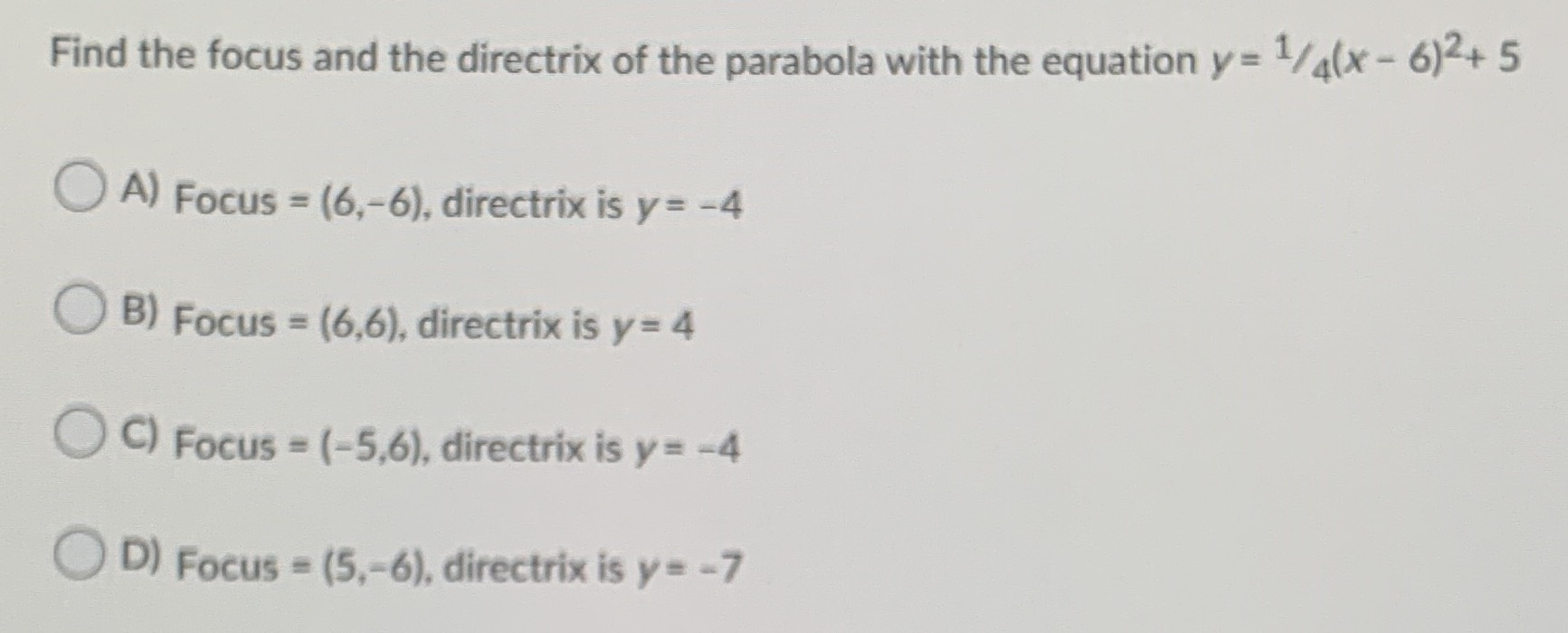### Still have math questions?

Algebra
QuestionFind the focus and the directrix of the parabola with the equation $$y = 1 / 4 ( x - 6 ) ^ { 2 } + 5$$

A) Focus $$= ( 6 , - 6 )$$ , directrix is $$y = - 4$$

B) Focus $$= ( 6,6 ) ,$$ directrix is $$y = 4$$

C) Focus $$= ( - 5,6 )$$ , directrix is $$y = - 4$$

D) Focus $$= ( 5 , - 6 )$$ , directrix is $$y = - 7$$